Willowshine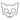鬼魂 (Q665)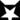无星之地 (Q635)[囚禁]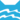河族 巫医河族 首席巫医
（继任自蛾翅 (Q2587)河族 巫医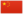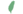小柳 Willowkit  小柳
 柳爪 Willowpaw  柳掌
 柳光 Willowshine  柳光

Q2852：河族母猫，自《新预言》系列登场的角色

## 细节

### 外貌

• 她是一只身形娇小:20章矫健:268的淡灰色:167虎斑:133母猫，:237有着光滑:268柔软:264的厚实皮毛:38和明亮的绿色眼睛。:133

### 作者声明

• WarriorCats.com的家谱树中，她是未知和藓毛的女儿，曙花的异父妹妹。
• 在《豹星的荣耀》出版前，Su[?]认为蛙跳是她的父亲；但由于该书描述了蛙跳的死亡时间点，而柳光在他死后两年才出生，因此他不可能是柳光的父亲。:19章

### 勘误

• 她曾被错误地描述为暗灰色:237和泛灰的白色。:167
• 她曾被错误地描述为蓝色眼睛，:4章并被描绘为暗蓝色眼睛。:75:137
• 她曾在三岁大时被错误地描述为“年幼得不能肩负起全部的巫医职务”。:4章

## 语录

—— 柳光在雾星面前维护蛾翅，《不为人知的故事》，第132-133页

—— 松鸦羽对柳光的想法，当蛾翅来雷族找他时，《暗夜密语》正体中文版，第18页

—— 蛾翅对松鸦羽，关于柳光对星族的忠诚，《群星之战》，第79页

## 登场书目

• 重现家园 (Q141)[配角][首次登场🐱]
• 黄昏战争 (Q143)[配角]
• 日落和平 (Q144)[配角]
• 预视力量 (Q152)[配角]
• 暗河汹涌 (Q153)[配角]
• 驱逐之战 (Q154)[配角]
• 天蚀遮月 (Q155)[配角]
• 暗夜长影 (Q156)[配角]
• 拂晓之光 (Q157)[配角]
• 第四学徒 (Q158)[猫物闲角]
• 战声渐近 (Q159)[配角]
• 暗夜密语 (Q160)[配角]
• 月光印记 (Q161)[配角]
• 武士归来 (Q162)[配角]
• 群星之战 (Q163)[配角]
• 学徒探索 (Q170)[配角]
• 雷影交加 (Q171)[配角]
• 天空破碎 (Q172)[配角]
• 极夜无光 (Q173)[配角]
• 烈焰焚河 (Q174)[视角人物]
• 风暴来袭 (Q175)[配角]
• 迷失群星 (Q3563)[配角]
• 静默冰融 (Q3579)[配角]
• 叠影重障 (Q3624)[配角]
• 暗由心生 (Q4183)[配角]
• 无星之地 (Q4385)[配角][死亡时刻✝]
• 迷雾明光 (Q4621)[配角]
• 河翻浪涌 (Q4643)[配角]
• 天穹将倾 (Q4799)[对话提及]
• 黑莓星的风暴 (Q182)[对话提及]
• 虎心的阴影 (Q185)[配角]
• 鸦羽的拷问 (Q186)[配角]
• 松鼠飞的希冀 (Q3561)[配角]
• 灰条的誓言 (Q4057)[猫物闲角]
• 冬青叶的故事 (Q187)[猫物闲角]
• 雾星的征兆 (Q188)[配角]
• 叶池的祈愿 (Q191)[猫物闲角]
• 鸽翅的沉默 (Q192)[猫物闲角]
• 褐皮的族群 (Q3566)[猫物闲角]
• 蛾翅的秘密 (Q3628)[配角]
• 黛西的至亲 (Q3629)[猫物闲角]
• 族群的猫 (Q200)[配角]
• 终极指南 (Q204)[配角]

## 脚注

1. 正体中文版中被译为“小柳”，但实际上原文是Willowpaw。

## 参考文献

这篇文章基于CC BY-SA 3.0许可使用了猫武士维基（英语）Willowshine一文中的部分内容。
1. 在同一份由维琪授意、Su所公布的黑森林名单中，官网家谱已将雪暴翅、亮花和沼云标注为星族成员，短尾标注为黑森林成员；鹿脚、百合须和缠果的死后去处则暂时没有更进一步的资讯。